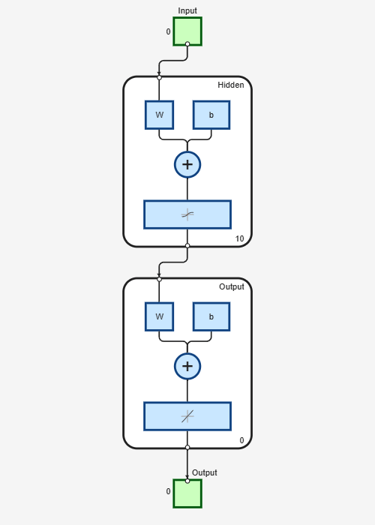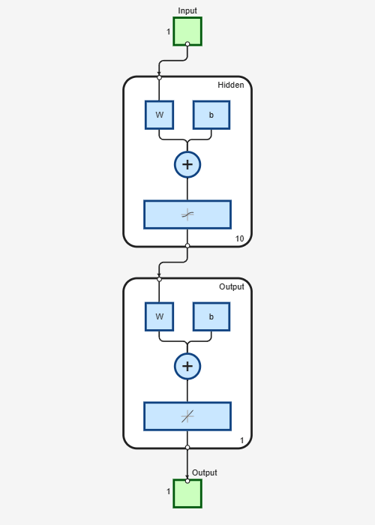# unconfigure

Unconfigure network inputs and outputs

## Syntax

```unconfigure(net) unconfigure(net, 'inputs', i) unconfigure(net, 'outputs', i) ```

## Description

`unconfigure(net)` returns a network with its input and output sizes set to 0, its input and output processing settings and related weight initialization settings set to values consistent with zero-sized signals. The new network will be ready to be reconfigured for data of the same or different dimensions than it was previously configured for.

`unconfigure(net, 'inputs', i)` unconfigures the inputs indicated by the indices `i`. If no indices are specified, all inputs are unconfigured.

`unconfigure(net, 'outputs', i)` unconfigures the outputs indicated by the indices `i`. If no indices are specified, all outputs are unconfigured.

## Examples

collapse all

This example shows how to configure a network for a simple fitting problem, and then unconfigure the network.

```[x,t] = simplefit_dataset; net = fitnet(10); view(net)``````net = configure(net,x,t); view(net)``````net = unconfigure(net); view(net)```## Version History

Introduced in R2010b